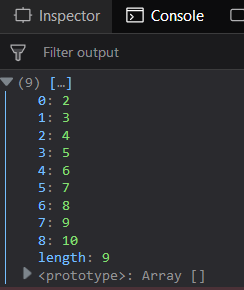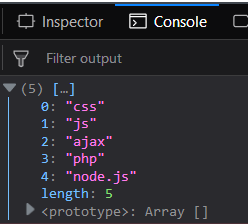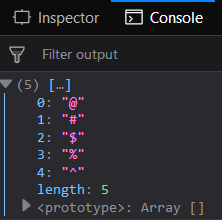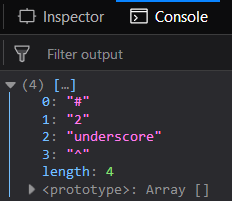Related Articles
Underscore.js | _.rest() with Examples
• Last Updated : 14 Feb, 2019

The Underscore.js is a JavaScript library that provides a lot of useful functions like the map, filter, invoke etc even without using any built-in objects.
The _.rest() is used to return the rest of the elements except the zeroth indexed element. Second parameter is used to start the search from the given indexed array. It is used to return all the array elements. When the element comes then only they are displayed except the first element of the index.

Syntax:

`_.rest( array, [index] ) `

Parameters: This function accepts two parameter which are listed below:

• array: This parameter is used to hold the array elements.
• index: This parameter is used to hold the index number.

Return value: It returns the rest elements in an array.

Passing a number array to the _.rest() function: The ._rest() function takes the element from the list one by one and displays the elements directly. Then number element is given to the _.rest() function. It ignores the first element from the array and then displays all the others.

Example:

 ` ` `<``html``> ` `    ``<``head``> ` `        ``<``script` `src` `=  ` `"https://cdnjs.cloudflare.com/ajax/libs/underscore.js/1.9.1/underscore-min.js"` `> ` `        `` ` `    `` ` `    ``<``body``> ` `        ``<``script` `type``=``"text/javascript"``> ` `            ``console.log(_.rest([1, 2, 3, 4, 5, 6, 7, 8, 9, 10])); ` `        `` ` `    `` ` `                     `

Output:Passing a word array to the _.rest() function: The ._rest() function takes the element from the list one by one and display the elements directly. It ignores whether the function is taking a numbered array or character array or any other. Then number element is given to the _.rest() function. It ignores the first element from the array and then displays all the others.

Example:

 ` ` `<``html``> ` `    ``<``head``> ` `        ``<``script` `src` `=  ` `"https://cdnjs.cloudflare.com/ajax/libs/underscore.js/1.9.1/underscore-min.js"` `> ` `        `` ` `    `` ` `    ``<``body``> ` `        ``<``script` `type``=``"text/javascript"``> ` `            ``console.log(_.rest(['html', 'css', 'js',  ` `                        ``'ajax', 'php', 'node.js'])); ` `        `` ` `    `` ` `                     `

Output:Passing a special character array to the _.rest() function: The ._rest() function takes the element from the list one by one and displays the elements directly inspite of the fact that it is containing special characters. Then the character element is given to the _.rest() function. It ignores the first element from the array and then displays all the others.

Example:

 ` ` `<``html``> ` `    ``<``head``> ` `        ``<``script` `src` `=  ` `"https://cdnjs.cloudflare.com/ajax/libs/underscore.js/1.9.1/underscore-min.js"` `> ` `        `` ` `    `` ` `    ``<``body``> ` `        ``<``script` `type``=``"text/javascript"``> ` `            ``console.log(_.rest(['!', '@', '#', '\$', '%', '^'])); ` `        `` ` `    `` ` `                     `

Output:Passing the second parameter to the _.rest() function: The second parameter is used to start displaying the elements from that index. It is used to ignore more than one array element as one element is ignored by default. It works the same for the rest of the elements.

Example:

 ` ` `<``html``> ` `    ``<``head``> ` `        ``<``script` `src` `=  ` `"https://cdnjs.cloudflare.com/ajax/libs/underscore.js/1.9.1/underscore-min.js"` `> ` `        `` ` `    `` ` `    ``<``body``> ` `        ``<``script` `type``=``"text/javascript"``> ` `            ``console.log(_.rest(['1', 'javascript', '#', ` `                            ``'2', 'underscore', '^'], 2)); ` `        `` ` `    `` ` `                     `

Output:Note: These commands will not work in Google console or in Firefox as for these additional files need to be added which they didn’t have added. So, add the given links to your HTML file and then run them.

 `<``script` `type``=``"text/javascript"` `src` `=   ` `"https://cdnjs.cloudflare.com/ajax/libs/underscore.js/1.9.1/underscore-min.js"``>   ` `  `My Personal Notes arrow_drop_up
Recommended Articles
Page :